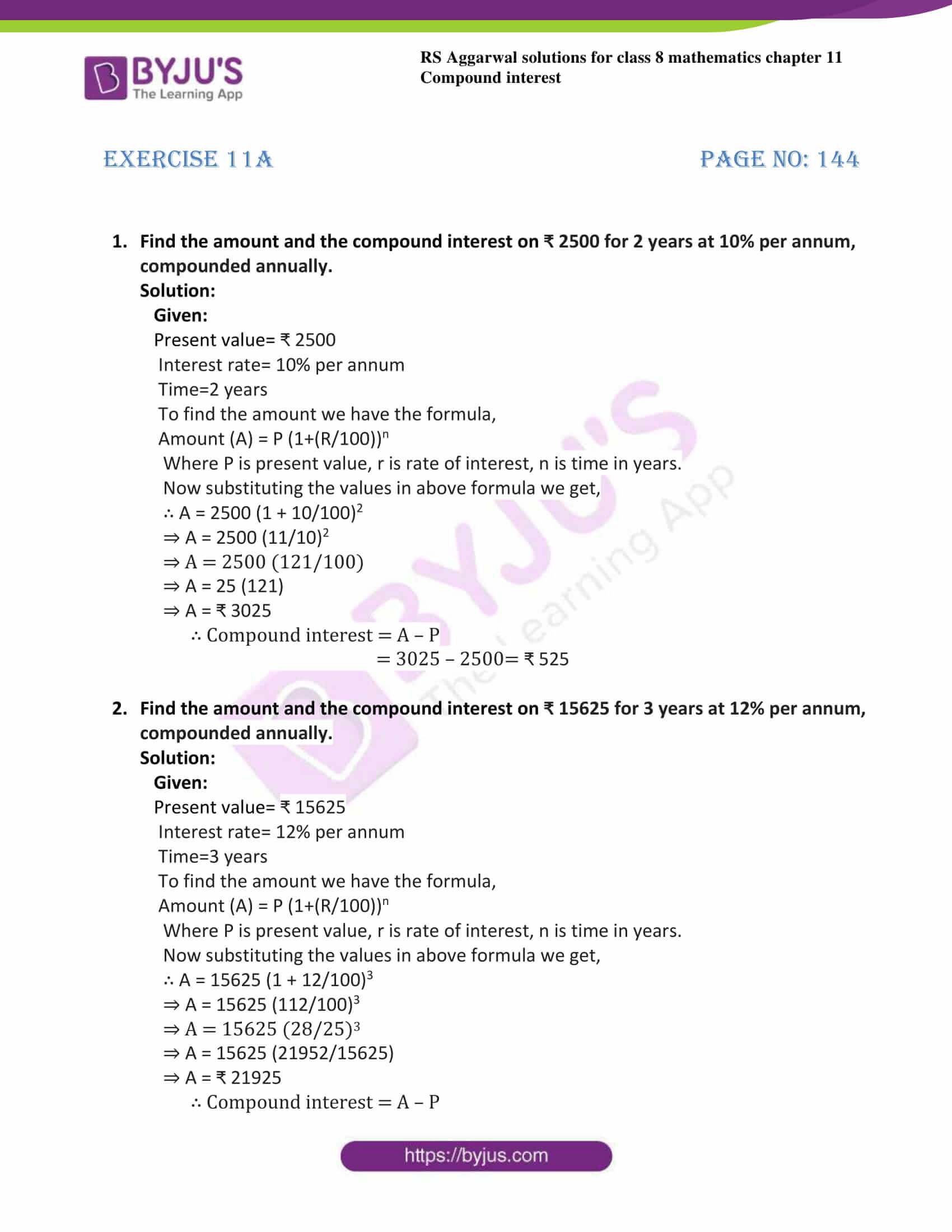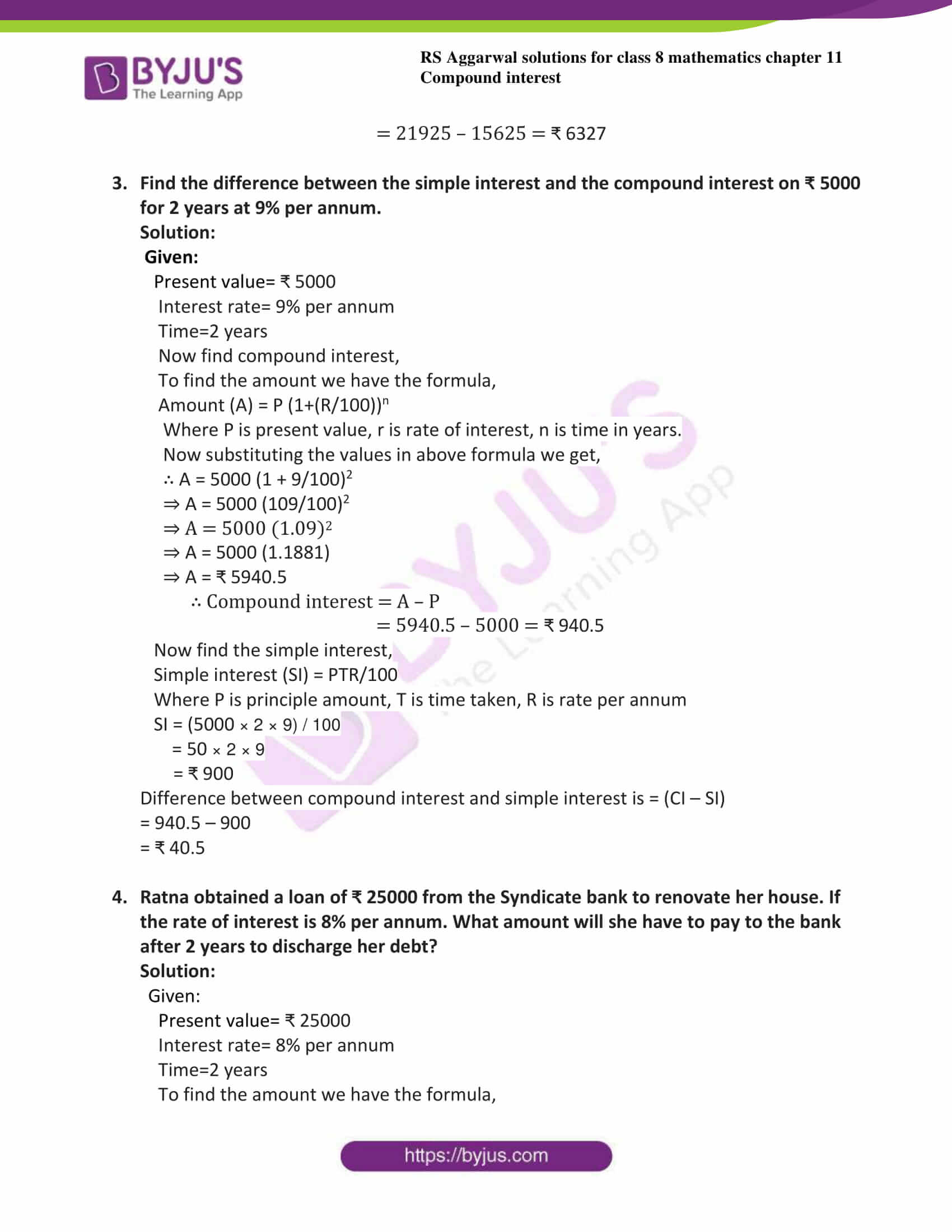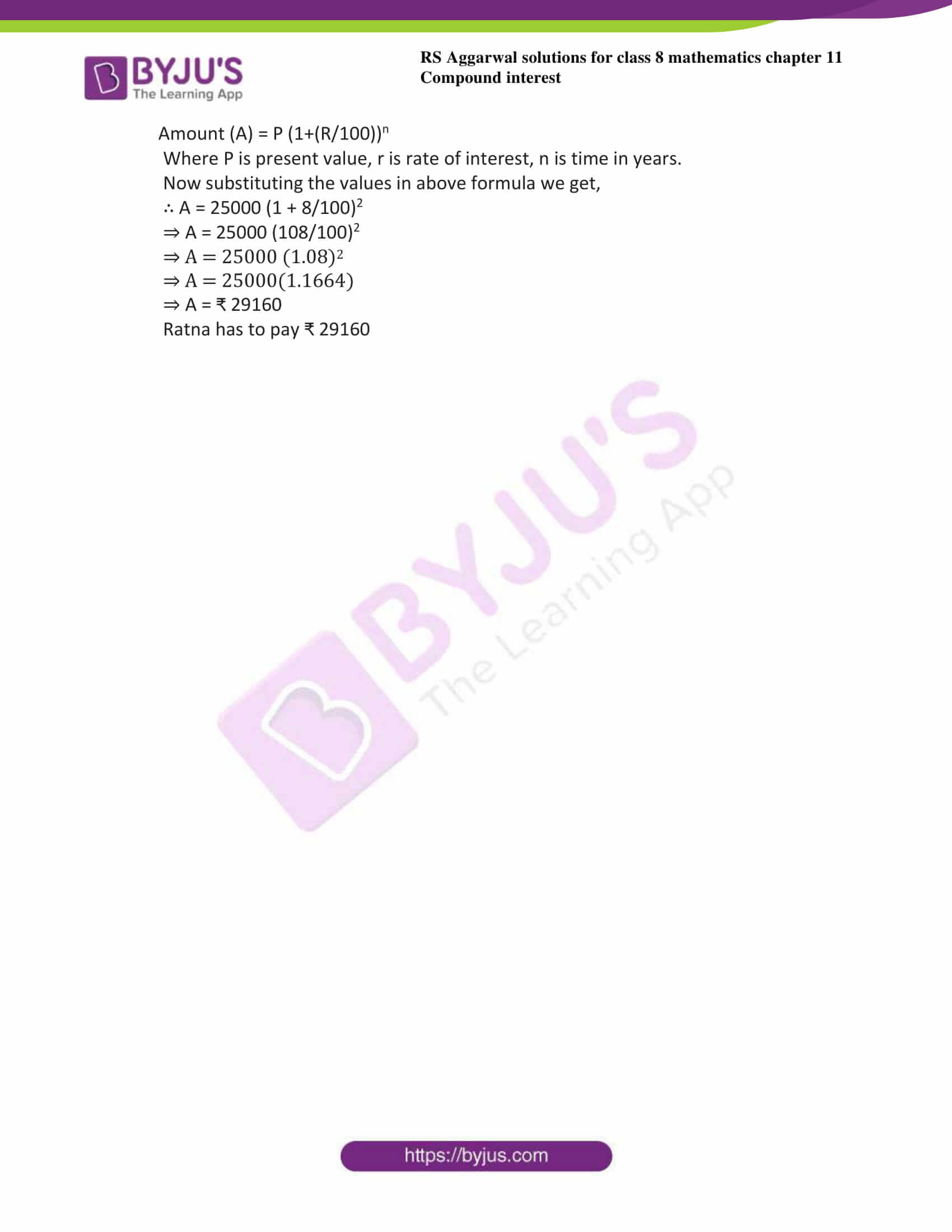# RS Aggarwal Solutions for Class 8 Maths Chapter 11 - Compound Interest Exercise 11A

Students can get the pdf of RS Aggarwal Solutions for the Exercise 11A of Class 8 Maths Chapter 11, Compound Interest from the following link. In this exercise, they earn knowledge about the compound interest which we use in our daily life. To get more in-depth knowledge about this, BYJU’S experts uniquely solved the exercise. As this is the first exercise of this chapter, it contains basic concepts related to the compound interest. By practising the RS Aggarwal Solutions for class 8, students will be able to grasp the concepts correctly. Hence, students whose aim is to score high in the examinations are directed to go through RS Aggarwal Solutions for Class 8.

## Download PDF of RS Aggarwal Solutions for Class 8 Maths Chapter 11 – Compound Interest Exercise 11A### Access answers to Maths RS Aggarwal Solutions for Class 8 Chapter 11 – Compound Interest Exercise 11A

1. Find the amount and the compound interest on ₹ 2500 for 2 years at 10% per annum, compounded annually.

Solution:

Given:

Present value= ₹ 2500

Interest rate= 10% per annum

Time=2 years

To find the amount we have the formula,

Amount (A) = P (1+(R/100))n

Where P is present value, r is rate of interest, n is time in years.

Now substituting the values in above formula we get,

∴ A = 2500 (1 + 10/100)2

⇒ A = 2500 (11/10)2

⇒ A = 2500 (121/100)

⇒ A = 25 (121)

⇒ A = ₹ 3025

∴ Compound interest = A – P

= 3025 – 2500= ₹ 525

2. Find the amount and the compound interest on ₹ 15625 for 3 years at 12% per annum, compounded annually.

Solution:

Given:

Present value= ₹ 15625

Interest rate= 12% per annum

Time=3 years

To find the amount we have the formula,

Amount (A) = P (1+(R/100))n

Where P is present value, r is rate of interest, n is time in years.

Now substituting the values in above formula we get,

∴ A = 15625 (1 + 12/100)3

⇒ A = 15625 (112/100)3

⇒ A = 15625 (28/25)3

⇒ A = 15625 (21952/15625)

⇒ A = ₹ 21925

∴ Compound interest = A – P

= 21925 – 15625 = ₹ 6327

3. Find the difference between the simple interest and the compound interest on ₹ 5000 for 2 years at 9% per annum.

Solution:

Given:

Present value= ₹ 5000

Interest rate= 9% per annum

Time=2 years

Now find compound interest,

To find the amount we have the formula,

Amount (A) = P (1+(R/100))n

Where P is present value, r is rate of interest, n is time in years.

Now substituting the values in above formula we get,

∴ A = 5000 (1 + 9/100)2

⇒ A = 5000 (109/100)2

⇒ A = 5000 (1.09)2

⇒ A = 5000 (1.1881)

⇒ A = ₹ 5940.5

∴ Compound interest = A – P

= 5940.5 – 5000 = ₹ 940.5

Now find the simple interest,

Simple interest (SI) = PTR/100

Where P is principle amount, T is time taken, R is rate per annum

SI = (5000 × 2 × 9) / 100

= 50 × 2 × 9

= ₹ 900

Difference between compound interest and simple interest is = (CI – SI)

= 940.5 – 900

= ₹ 40.5

4. Ratna obtained a loan of ₹ 25000 from the Syndicate bank to renovate her house. If the rate of interest is 8% per annum. What amount will she have to pay to the bank after 2 years to discharge her debt?

Solution:

Given:

Present value= ₹ 25000

Interest rate= 8% per annum

Time=2 years

To find the amount we have the formula,

Amount (A) = P (1+(R/100))n

Where P is present value, r is rate of interest, n is time in years.

Now substituting the values in above formula we get,

∴ A = 25000 (1 + 8/100)2

⇒ A = 25000 (108/100)2

⇒ A = 25000 (1.08)2

⇒ A = 25000(1.1664)

⇒ A = ₹ 29160

Ratna has to pay ₹ 29160

## RS Aggarwal Solutions for Class 8 Maths Chapter 11 – Compound Interest Exercise 11A

Exercise 11A of RS Aggarwal Solutions for Chapter 11, Compound Interest deals with the topics related to compound interest and methods of solving compound interest. Some of the topics focused prior to exercise 11A include the following.

• Definition of compound interest
• To find compound interest when interest is compounded annually
• To find compound interest when interest is compounded half-yearly

The RS Aggarwal Solutions can help the students in exercising and learning each and every concept as it provides solutions to all questions asked in the RS Aggarwal textbook. Those who aim to score high in the Maths of class 8 are advised to practice all the questions present in RS Aggarwal as many times as possible.